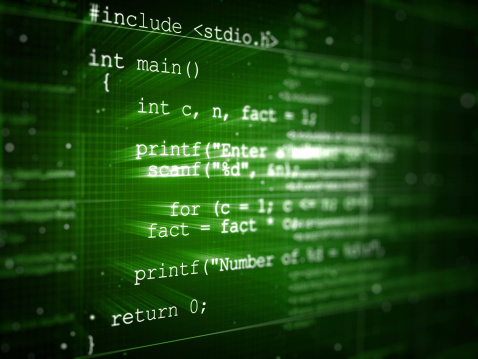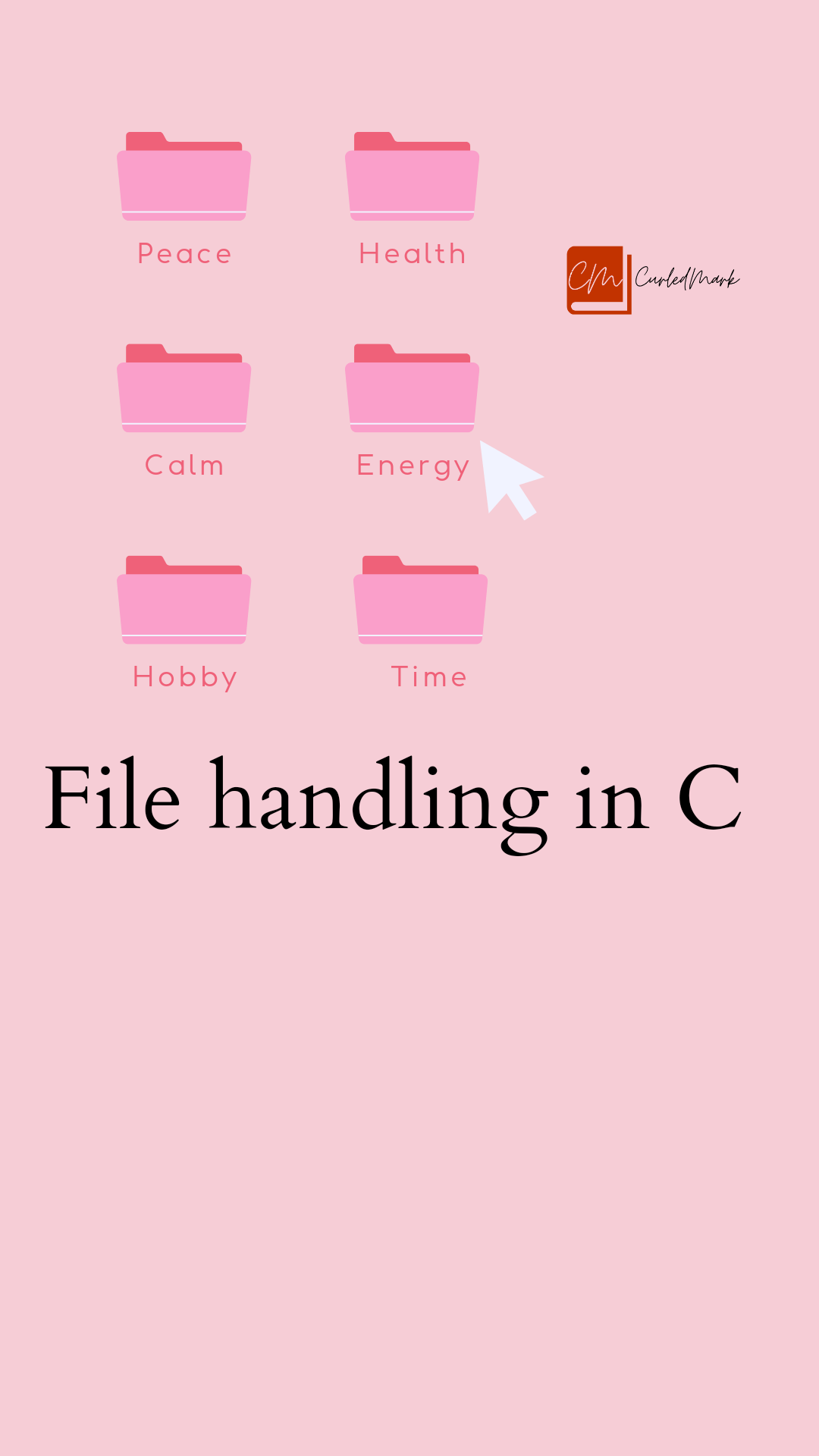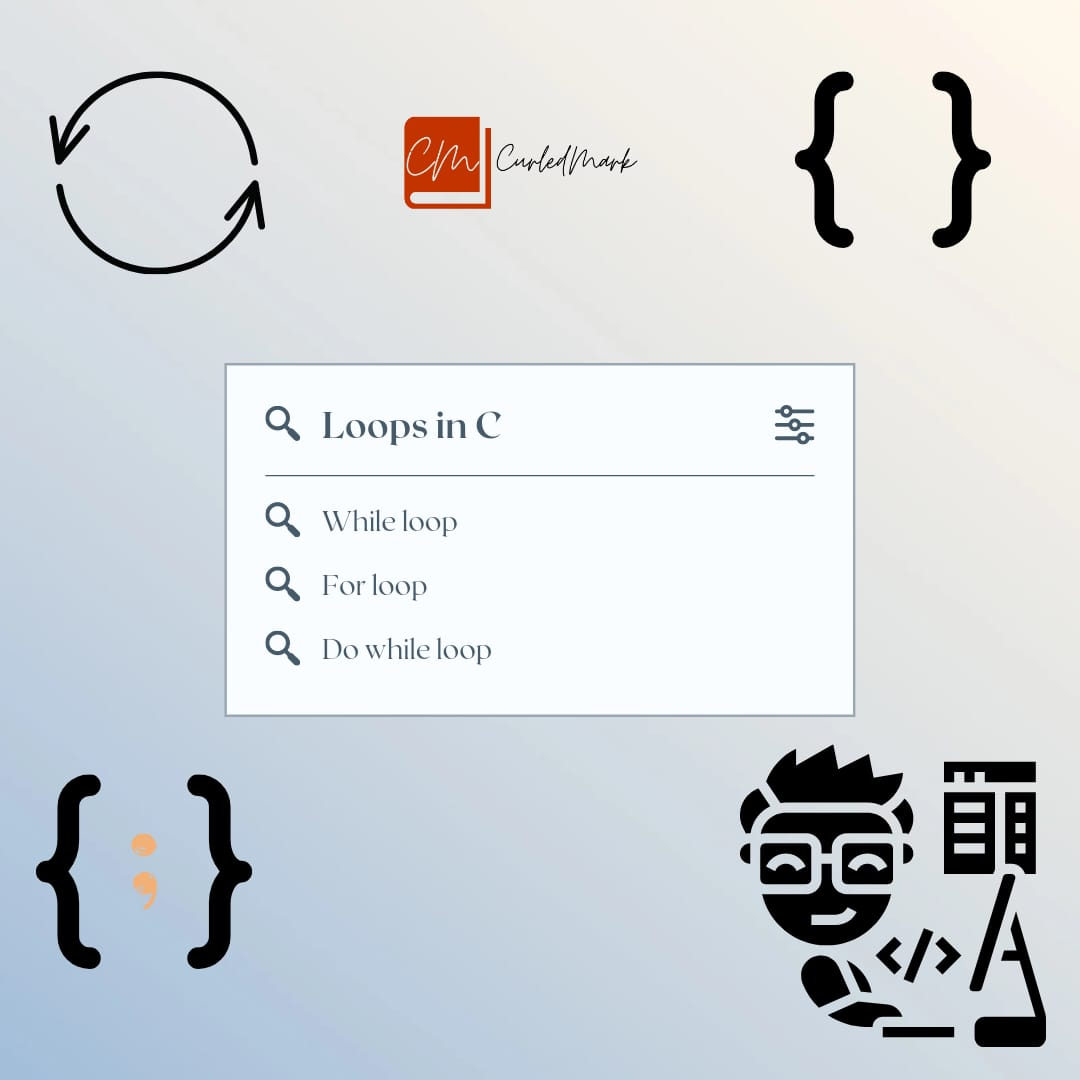January 30, 2023Program Code

# Looping and printing number series in C

Computers are very good at performing repetitive tasks very quickly. In this section, we will learn how to make computer repeat actions either a specified number of times or until some stopping condition is met.

## while Loops ( Condition-Controlled Loops ) in C

• Both while loops and do-while loops ( see below ) are condition-controlled, meaning that they continue to loop until some condition is met.
• Both while and do-while loops alternate between performing actions and testing for the stopping condition.
• While loops check for the stopping condition first, and may not execute the body of the loop at all if the condition is initially false.

For the looping construct, there are different types of keywords. One of them is the While loop. The Syntax for this is:

`While(condition)`

`{`

`//definition`

`}`

If the condition is true, the control will go inside the loop and work. After then the control will go back to the While.## Print 1,2,3,4,5 series in C

`#include<stdio.h>`

`int main()`

`{`

`int x;`

`x=1;`

`While(x<=5)`

`{`

`Printf("%d\n",x);`

`x++;`

`}`

`return 0;`

`} `

Output:

1

2

3

4

5

## Print the 1,2,4,7,11 series in C

`#include<stdio.h>`

`int main()`

`{`

`int x,y;`

`x=1; y=1;`

`While(x<=5)`

`{`

`Printf("%d\n",y);`

`y=y+x;`

`x++;`

`}`

`return 0;`

`}`

Output:

1

2

4

7

11

## Print the 1,2,6,24,120 series in C

` #include<stdio.h>`

`int main()`

`{`

`int x=1,y=1;`

`While(x<=5)`

`{`

`y=y*x;`

`Printf("%d\n",y);`

`x++;`

`}`

`return 0;`

`}`

## Print the Fibonacci series in C

`#include<stdio.h>`

`int main()`

`{`

`int x,y,z;`

`x=1,y=1;`

`While(x<=34)`

`{`

`Printf("%d\n",x);`

`z=x+y;`

`x=y;`

`y=z;`

`}`

`return 0;`

`}`

Operators and accepting input from Keyboard in C Programming

Get Oracle Foundations Associate Certified

THE LONG WAY TO A SMALL, ANGRY PLANET BOOK SUMMARY

6 Time Management Tips to Get More Done | Brian Tracy

AS A MAN THINKETH BOOK SUMMARY

#### You may have missed#### RIYA SHUKLA, DIVYANSH DWVEDI & CHITRANSH RAAJ TO SHARE SCREEN IN FILM PULLU